# Overview

This is a demonstration of regularized mediation analysis methods based on penalized structural equation modeling. The original version (version 1.x) on CRAN was for structural equations with multiple mediators between a single exposure variable and a single outcome variable. The penalized model implemented in the regmed functions is based on sparse group lasso in which the pair of parameters alpha and beta are considered a group; alpha is the effect of exposure on a mediator and beta is the effect of that mediator on the outcome. Version 2.x and later include now include computationally efficient models that allow for multiple exposures, multiple mediators, and multiple outcomes. The penalized model for this multivariate situation, implemented in functions denoted mvregmed, uses a lasso (L1) type of penalty for all parameters: alpha that link exposures with mediators; beta that link mediators with outcomes; delta that link exposures directly with outcomes.

The default way of analyzing the data is to center and scale all x, mediator, and y variables, so each column of the x, mediator, and y variables has mean 0 and variance 1. This default approach is recommended, because it places all variables on the same scale. If adjustment for extraneous covariates is needed, regression of any of the variables on covaraites should be performed prior to use of regmed or mvregmed, and the residuals from these regression models can be used as input variables to the methods of regmed or mvregmed. When standardized varibles are used, the penalized structural equation models are modeling the correlations of variables.

## Simulated dataset

The simulated data set (named “medsim”) contains 100 subjects with 10 exposure “x” variables, 200 mediator “med” variables, and 2 outcome “y” variables.

data(medsim)

# regmed: Mediation model for 1 exposure, multiple mediators, and 1 outcome variable

This section focuses on the original version of regmed that handles a single exposure and a single outcome. The user user-level functions for single outcome/exposure are:

• regmed.prefilter() pre-filters mediators if there are more than the number of subjects
• regmed.grid() penalized model for grid of lambdas
• regmed.grid.bestfit() get best fit model from grid of fits
• regmed.fit() fit penalized model with specified lambda
• plot.regmed.grid() plot results of regmed.grid
• regmed.edges() create edges from a fit of a model for use in plotting and model fit by lavaan
• plot.regmed.edges() plot directed graph based on edges: exposure -> mediator -> outcome
• regmed.lavaan.model() setup model to estimate unpenalized parameters by lavaann sem function
• regmed.lavaan.dat() setup data to input tolavaann sem function
• summary.lavaan() summarize lavaan sem model fit

## Pre-filter mediators

If the number of mediators exceeds the sample size, model fitting can become unstable. To reduce the number of mediators to fit, the regmed.prefilter uses sure independence screening (Fan & Lv, 2008) to reduce the number of potential mediator. This is based on ranking marginal correlations and then selecting the highest ranked values such that the number of parameters is less than the sample size. Because mediation depends on the two correlations, $$cor(x,med)$$ and $$cor(med, y)$$ we rank the absolute values of their products, $$|cor(x, med) * cor(med, y)|$$, and choose the highest k ranked values to determine which potential mediators to include in penalized mediation models. If k is not specified, the default value of k is n/2, where n is the sample size, because each mediator results in two parameters alpha and beta.

We choose k=10 mediators to select, to speed calculations solely for demonstration purposes. Also, we select the first exposure variable and the first outcome variable to demonstrate methods for regmed. The function regmed.prefilter returns a list of x, mediator, and y, with each of these variables centered and scaled by default, and accounts for missing data by subsetting to subjects that are not missing any of the variables.

dat.filter <- regmed.prefilter(x[, 1], med, y[, 1], k = 10)

## Grid of lambda penalties

To fit a series of models over a grid of penalty “lambda” values, it is necessary to define a vector of lambda values. It is best to arrange this vector from largest to smallest lambda values to assure that the largest lambda (first value in the vector) results in no selected parameters (alpha, beta, delta), and the smallest lambda (last value in the vector) selects multiple parameters, and that a lambda between the largest and smallest results in a minimum Bayesian Information Criterion (BIC). Starting with a large lambda and then decreasing lambda values is best, because this provides a “warm start” for each subsequent fit, with final fitted parameter values for a specific lambda used as initial values for the next lambda value. The demonstration below extracts variables that have been processed by regmed.prefilter.

## Fit regmed for grid of lambda penalties

lambda.grid <- seq(from = 0.4, to = 0.01, by = -0.01)

x1 <- dat.filter$x y1 <- dat.filter$y
med <- dat.filter$mediator fit.grid <- regmed.grid(x1, med, y1, lambda.grid, frac.lasso = 0.8) fitting grid lambda [ 1 ] = 0.4 fitting grid lambda [ 2 ] = 0.39 fitting grid lambda [ 3 ] = 0.38 fitting grid lambda [ 4 ] = 0.37 fitting grid lambda [ 5 ] = 0.36 fitting grid lambda [ 6 ] = 0.35 fitting grid lambda [ 7 ] = 0.34 fitting grid lambda [ 8 ] = 0.33 fitting grid lambda [ 9 ] = 0.32 fitting grid lambda [ 10 ] = 0.31 fitting grid lambda [ 11 ] = 0.3 fitting grid lambda [ 12 ] = 0.29 fitting grid lambda [ 13 ] = 0.28 fitting grid lambda [ 14 ] = 0.27 fitting grid lambda [ 15 ] = 0.26 fitting grid lambda [ 16 ] = 0.25 fitting grid lambda [ 17 ] = 0.24 fitting grid lambda [ 18 ] = 0.23 fitting grid lambda [ 19 ] = 0.22 fitting grid lambda [ 20 ] = 0.21 fitting grid lambda [ 21 ] = 0.2 fitting grid lambda [ 22 ] = 0.19 fitting grid lambda [ 23 ] = 0.18 fitting grid lambda [ 24 ] = 0.17 fitting grid lambda [ 25 ] = 0.16 fitting grid lambda [ 26 ] = 0.15 fitting grid lambda [ 27 ] = 0.14 fitting grid lambda [ 28 ] = 0.13 fitting grid lambda [ 29 ] = 0.12 fitting grid lambda [ 30 ] = 0.11 fitting grid lambda [ 31 ] = 0.1 fitting grid lambda [ 32 ] = 0.09 fitting grid lambda [ 33 ] = 0.08 fitting grid lambda [ 34 ] = 0.07 fitting grid lambda [ 35 ] = 0.06 fitting grid lambda [ 36 ] = 0.05 fitting grid lambda [ 37 ] = 0.04 fitting grid lambda [ 38 ] = 0.03 fitting grid lambda [ 39 ] = 0.02 fitting grid lambda [ 40 ] = 0.01  The plot method for regmed.grid gives two figures that represent the size of the coefficients over the grid of lambdas, and the BIC by by the grid of lambdas. plot.regmed.grid(fit.grid)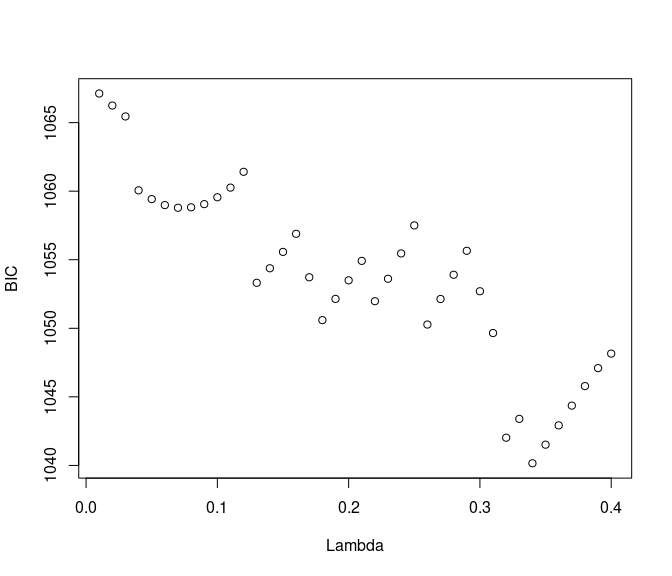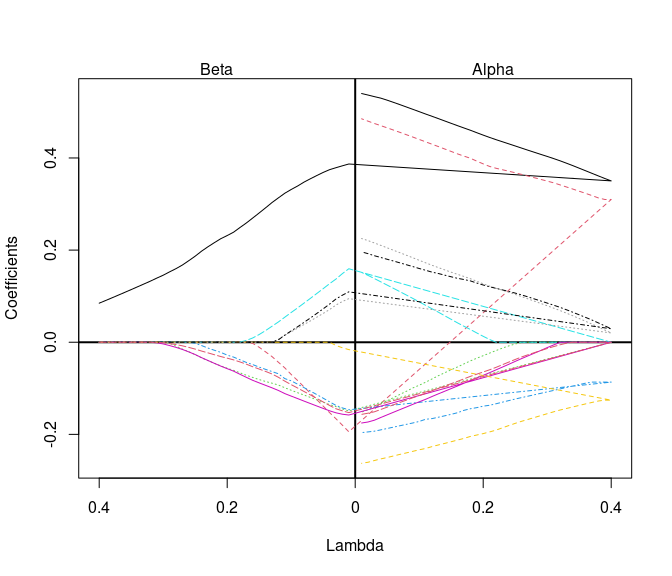## Select best model from grid by minimum BIC fit.best <- regmed.grid.bestfit(fit.grid) summary(fit.best) Call: NULL Coefficients: alpha beta alpha*beta med.1 0.38341929 0.1203989 0.04616325 med.2 0.33255320 0.0000000 0.00000000 med.74 0.00000000 0.0000000 0.00000000 med.88 -0.09504961 0.0000000 0.00000000 med.90 0.00000000 0.0000000 0.00000000 med.99 0.00000000 0.0000000 0.00000000 med.112 -0.14050034 0.0000000 0.00000000 med.129 0.05748298 0.0000000 0.00000000 med.133 0.06508961 0.0000000 0.00000000 med.178 0.00000000 0.0000000 0.00000000 sum of alpha*beta = 0.04616325 delta = 0.5046204 sum of delta + alpha*beta = 0.5507836 var(x) = 1 var(y) = 1.102819  ## Fit single regmed model with user-specified lambda If user prefers to specify a lambda penalty and not search through a grid, the function regmed.fit is available. fit.single <- regmed.fit(x1, med, y1, lambda = 0.3, frac.lasso = 0.8) summary(fit.single) Call: regmed.fit(x = x1, mediator = med, y = y1, lambda = 0.3, frac.lasso = 0.8) Coefficients: alpha beta alpha*beta med.1 0.40109014 0.126420020 5.070582e-02 med.2 0.35582882 0.000000000 0.000000e+00 med.74 0.00000000 0.000000000 0.000000e+00 med.88 -0.13906482 0.000000000 0.000000e+00 med.90 0.00000000 0.000000000 0.000000e+00 med.99 -0.01045770 -0.001413514 1.478211e-05 med.112 -0.17587944 0.000000000 0.000000e+00 med.129 0.07206755 0.000000000 0.000000e+00 med.133 0.07530145 0.000000000 0.000000e+00 med.178 -0.01914775 0.000000000 0.000000e+00 sum of alpha*beta = 0.05072061 delta = 0.5386689 sum of delta + alpha*beta = 0.5893895 var(x) = 1 var(y) = 1.116486  ## Plot directed graph of fit model The demonstration below shows how to determined edges, where an edge is defined by vertex-1 pointing to vertex-2, and the vertices are the variables selected in a model. For example, if alpha and beta are both estimated to be non-zero, then x -> med and med -> y. The function regmed.edges has an option to choose how vertices and edges are selected. By type=“mediators”, vertices and edges are selected only of the product $$alpha * beta$$ is non-zero, because this is required for a mediator to be truely mediating. By specifying type = “any”, all vertices and edges that represent non-zero parameters are selected. For example, if alpha is non-zero, and beta is zero, the edge x -> med is selected, but the edge med -> y is not selected. To determine if terms are zero, a threshold variable eps is used (see help for regmed.edges). For fit.best, the summary above shows that only med.1 is selected as a mediator, because $$|alpha * beta| > eps$$. In this case, choosing type=“mediators” selects only med.1 to be included in edges. In contrast, choosing type=“mediators” for fit.best results in including med.2 as an edge, even thouth it is not a mediator. edges.med <- regmed.edges(fit.best, type = "mediators") plot.regmed.edges(edges.med)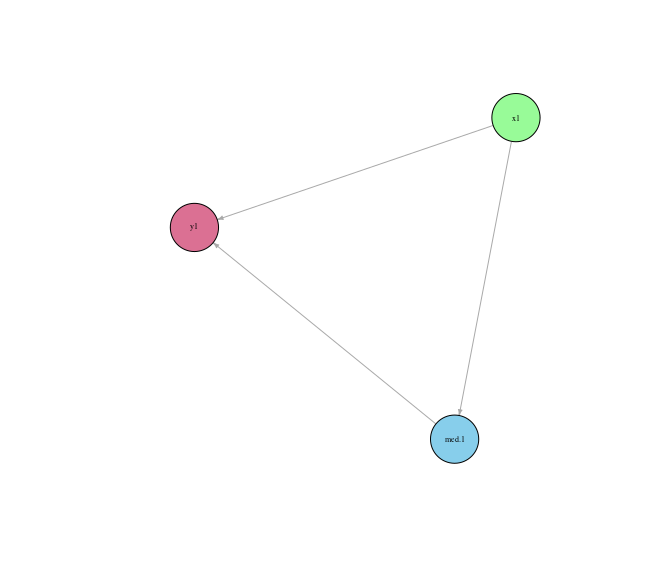edges.any <- regmed.edges(fit.best, type = "any") plot.regmed.edges(edges.any)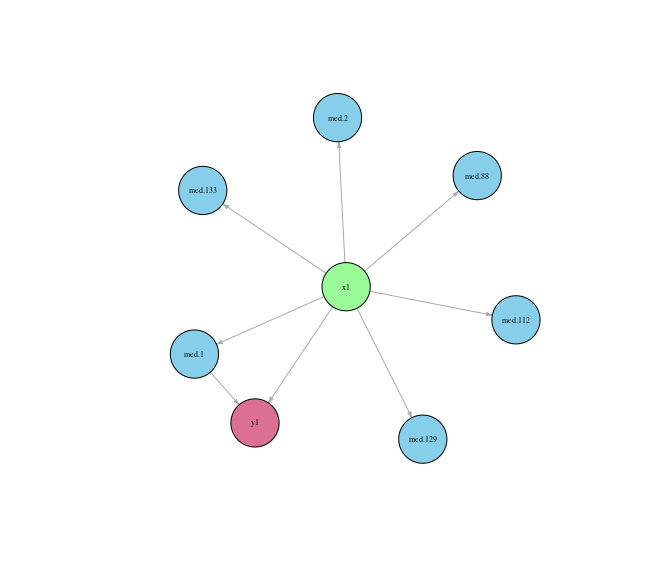## Lavaan sem: Estimate parameters of selected model without imposing penalty Because penalized models can overly shink parameter estimates towards zero, it can be beneficial to refit the selected model without penalties. The model without penalties is a starndard structural equation model, which can be fit with the function sem from the package lavaan. To facilitate this step, the function regmed.lavaan.model uses the edges and fit of a model to create a text string that represents the model syntax for sem. This function abides by our assumption that the residual covariances between x and med, between x and y, and between med and y, are all zero. It also assumes that the covariances of the mediators, a matrix in the fit of a regmed object, are fixed in the sem model fitting. The demonstration below shows how to setup the models for sem as well as a data set. The function regmed.lavaan.dat assures that x, med, y, are centered and scaled and subset to subjects without any missing data. mod.best <- regmed.lavaan.model(edges.med, fit.best) mod.any <- regmed.lavaan.model(edges.any, fit.best) dat <- regmed.lavaan.dat(x1, med, y1) The demonstration below shows how to use lavaan sem to fit specified models and view resuts fit.lav.med <- sem(model = mod.best, data = dat) summary.lavaan(fit.lav.med)  lhs op rhs est se z pvalue ci.lower ci.upper 1 med.1 ~ x1 0.551 0.084 6.577 0.000 0.387 0.716 2 y1 ~ med.1 0.293 0.093 3.147 0.002 0.110 0.475 3 y1 ~ x1 0.415 0.093 4.445 0.000 0.232 0.598 4 y1 ~~ y1 0.602 0.085 7.071 0.000 0.435 0.769 fit.lav.any <- sem(model = mod.any, data = dat) summary.lavaan(fit.lav.any)  lhs op rhs est se z pvalue ci.lower ci.upper 1 med.1 ~ x1 0.551 0.084 6.577 0.000 0.387 0.716 2 med.2 ~ x1 0.489 0.088 5.576 0.000 0.317 0.661 3 med.88 ~ x1 -0.197 0.099 -2.002 0.045 -0.390 -0.004 4 med.112 ~ x1 -0.264 0.097 -2.723 0.006 -0.454 -0.074 5 med.129 ~ x1 0.232 0.098 2.369 0.018 0.040 0.423 6 med.133 ~ x1 0.200 0.098 2.032 0.042 0.007 0.393 7 y1 ~ med.1 0.293 0.093 3.147 0.002 0.110 0.475 8 y1 ~ x1 0.415 0.093 4.445 0.000 0.232 0.598 9 y1 ~~ y1 0.602 0.085 7.071 0.000 0.435 0.769 # mvregmed : Mediation model for multiple exposures, multiple mediators, and multiple outcome variables New in version 2.0 is the multivariate version mvregmed, mv for multivariate. The user-level functions available for these are listed as follows: • mvregmed.grid() penalized model for grid of lambdas • mvregmed.grid.bestfit() get best fit mvregmed model from grid of fits • mvregmed.fit() fit penalized mvregmed model with specified lambda • plot.mvregmed.grid() plot results of mvregmed.grid • plot.mvregmed() plot results of a single mv regmed model fit, from either mvregmed.fit or mvregmed.grid.bestfit • mvregmed.edges() create edges from a fit of a model for use in plotting and model fit by lavaan • plot.mvregmed.edges() plot directed graph based on edges: exposure -> mediator -> outcome • regmed.lavaan.model() setup model to estimate unpenalized parameters by lavaann sem function • regmed.lavaan.dat() setup data to input to lavaann sem function • summary.lavaan() summarize lavaan sem model fit, which applies to both regmed.fit and mvregmed.fit runs in the lavaan::sem, because the outputs are the same. ## Fit mvregmed for a grid for lambda penalties With a specified lambda.grid, fit a grid of models over the multiple exposures and mediators. We see in the summary and the plot that a lambda around 0.10 provides the best model. fit.grid <- mvregmed.grid(x, med, y, lambda.grid) fitting grid lambda [ 1 ] = 0.4 fitting grid lambda [ 2 ] = 0.39 fitting grid lambda [ 3 ] = 0.38 fitting grid lambda [ 4 ] = 0.37 fitting grid lambda [ 5 ] = 0.36 fitting grid lambda [ 6 ] = 0.35 fitting grid lambda [ 7 ] = 0.34 fitting grid lambda [ 8 ] = 0.33 fitting grid lambda [ 9 ] = 0.32 fitting grid lambda [ 10 ] = 0.31 fitting grid lambda [ 11 ] = 0.3 fitting grid lambda [ 12 ] = 0.29 fitting grid lambda [ 13 ] = 0.28 fitting grid lambda [ 14 ] = 0.27 fitting grid lambda [ 15 ] = 0.26 fitting grid lambda [ 16 ] = 0.25 fitting grid lambda [ 17 ] = 0.24 fitting grid lambda [ 18 ] = 0.23 fitting grid lambda [ 19 ] = 0.22 fitting grid lambda [ 20 ] = 0.21 fitting grid lambda [ 21 ] = 0.2 fitting grid lambda [ 22 ] = 0.19 fitting grid lambda [ 23 ] = 0.18 fitting grid lambda [ 24 ] = 0.17 fitting grid lambda [ 25 ] = 0.16 fitting grid lambda [ 26 ] = 0.15 fitting grid lambda [ 27 ] = 0.14 fitting grid lambda [ 28 ] = 0.13 fitting grid lambda [ 29 ] = 0.12 fitting grid lambda [ 30 ] = 0.11 fitting grid lambda [ 31 ] = 0.1 fitting grid lambda [ 32 ] = 0.09 fitting grid lambda [ 33 ] = 0.08 fitting grid lambda [ 34 ] = 0.07 fitting grid lambda [ 35 ] = 0.06 fitting grid lambda [ 36 ] = 0.05 fitting grid lambda [ 37 ] = 0.04 fitting grid lambda [ 38 ] = 0.03 fitting grid lambda [ 39 ] = 0.02 fitting grid lambda [ 40 ] = 0.01  summary(fit.grid)  lambda converge iter df df.alpha df.beta df.delta df.vary bic 1 0.40 TRUE 1 3 0 0 0 3 1654.792 2 0.39 TRUE 1 3 0 0 0 3 1654.792 3 0.38 TRUE 1 3 0 0 0 3 1654.792 4 0.37 TRUE 1 3 0 0 0 3 1654.792 5 0.36 TRUE 1 3 0 0 0 3 1654.792 6 0.35 TRUE 1 3 0 0 0 3 1654.792 7 0.34 TRUE 2 4 0 0 1 3 1658.532 8 0.33 TRUE 16 4 0 0 1 3 1654.905 9 0.32 TRUE 20 4 0 0 1 3 1650.588 10 0.31 TRUE 17 4 0 0 1 3 1646.938 11 0.30 TRUE 20 6 1 1 1 3 1648.360 12 0.29 TRUE 18 6 1 1 1 3 1640.782 13 0.28 TRUE 15 7 2 1 1 3 1637.233 14 0.27 TRUE 13 7 2 1 1 3 1628.011 15 0.26 TRUE 11 7 2 1 1 3 1619.421 16 0.25 TRUE 10 8 2 2 1 3 1615.833 17 0.24 TRUE 13 8 2 2 1 3 1606.546 18 0.23 TRUE 13 8 2 2 1 3 1597.676 19 0.22 TRUE 12 8 2 2 1 3 1589.353 20 0.21 TRUE 11 8 2 2 1 3 1581.557 21 0.20 TRUE 10 8 2 2 1 3 1574.278 22 0.19 TRUE 9 8 2 2 1 3 1567.483 23 0.18 TRUE 9 9 2 3 1 3 1565.120 24 0.17 TRUE 10 10 2 4 1 3 1562.287 25 0.16 TRUE 10 12 3 5 1 3 1562.820 26 0.15 TRUE 10 14 4 5 2 3 1562.427 27 0.14 TRUE 9 16 5 6 2 3 1562.163 28 0.13 TRUE 9 19 8 6 2 3 1564.848 29 0.12 TRUE 8 20 9 6 2 3 1557.575 30 0.11 TRUE 8 23 10 6 4 3 1559.310 31 0.10 TRUE 9 24 10 7 4 3 1551.370 32 0.09 TRUE 7 27 11 8 5 3 1553.866 33 0.08 TRUE 8 30 13 9 5 3 1556.457 34 0.07 TRUE 13 38 16 11 8 3 1578.555 35 0.06 TRUE 14 40 16 13 8 3 1572.406 36 0.05 TRUE 16 48 21 14 10 3 1593.056 37 0.04 TRUE 14 59 30 14 12 3 1622.029 38 0.03 TRUE 13 74 43 14 14 3 1661.687 39 0.02 TRUE 13 92 56 16 17 3 1712.467 40 0.01 TRUE 17 120 82 18 17 3 1809.103 plot(fit.grid)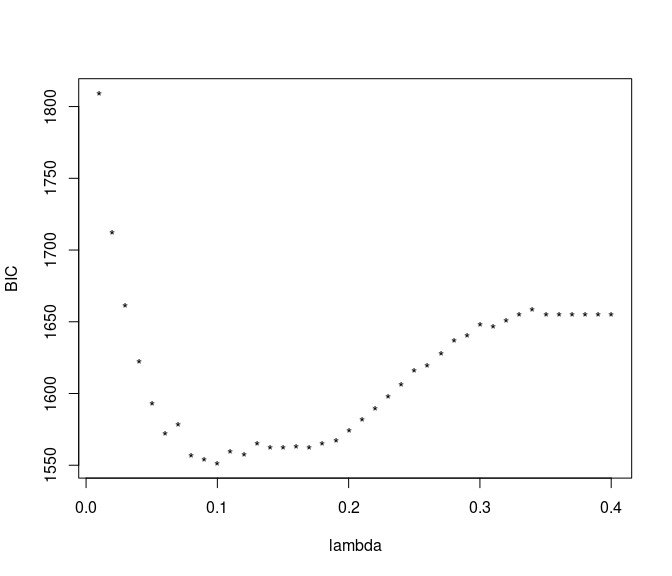## Select best model from grid based on minimum BIC Choose best fit model from grid based on min BIC. mvfit.best <- mvregmed.grid.bestfit(fit.grid) Instead of examining all contents of a model, use summary to view main non-zero parameters from alpha, beta, and delta. summary(mvfit.best) === alpha parameter estimates === mediator x alpha 1 med.1 x.1 0.35439717 2 med.1 x.5 0.05232707 3 med.2 x.1 0.30707983 4 med.88 x.1 -0.08543541 5 med.112 x.1 -0.09557552 6 med.112 x.2 -0.03397326 7 med.129 x.1 0.04703450 8 med.129 x.2 0.03646951 9 med.133 x.1 0.02059390 10 med.133 x.5 0.06844645 === beta parameter estimates === y mediator beta 1 y.1 med.1 0.27605124 2 y.1 med.74 -0.07297674 3 y.1 med.88 -0.04104814 4 y.1 med.90 0.01331543 5 y.1 med.99 -0.09497897 6 y.1 med.178 -0.05289736 7 y.2 med.2 0.26795394 === delta parameter estimates === y x delta 1 y.1 x.1 0.27186184 2 y.1 x.5 0.01699286 3 y.1 x.8 0.05065293 4 y.1 x.10 -0.04389265 ## Fit single mvregmed model with user-specified lambda Below shows how to fit a single model, and what happens with summary when all alpha, beta, and delta are 0. mvfit <- mvregmed.fit(x, med, y, lambda = 0.5) summary(mvfit) Warning in summary.mvregmed(mvfit): all alpha, beta, delta are zero NULL ## Plot directed graph of fit model After edges are created, we use the pakage igraph to create plots. Because igraph generates graphs based on random seed, it is imporatnt to set a seed to assure the plot can be recreated. We provide the simple function plot.mvregmed.edges to create a basic plot. ## get vertices and edges of graph mvfit.edges <- mvregmed.edges(mvfit.best, eps = 0.01) plot.mvregmed.edges(mvfit.edges, x.color = "palegreen", y.color = "palevioletred", med.color = "skyblue", v.size = 20, seed = 3)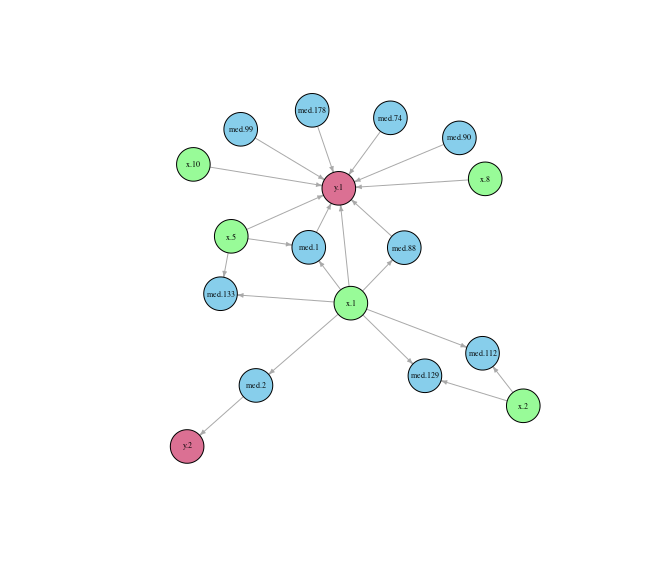To have more control of igraph attributes, we demonstrate below how to use our function mvregmed.graph.attributes to set up initial graph attributes, and then set a variety of igraph attributes in a standard plot function. See igraph for more plot attributes. gr <- regmed:::mvregmed.graph.attributes(mvfit.edges, x.color = "palegreen", y.color = "palevioletred", med.color = "skyblue", v.size = 30) set.seed(3) plot(gr$gr, vertex.size = gr$vsize, vertex.color = gr$vcol, vertex.label.font = 1, vertex.label.color = "black",
vertex.label.cex = 0.5, edge.arrow.mode = ">", edge.arrow.size = 0.3)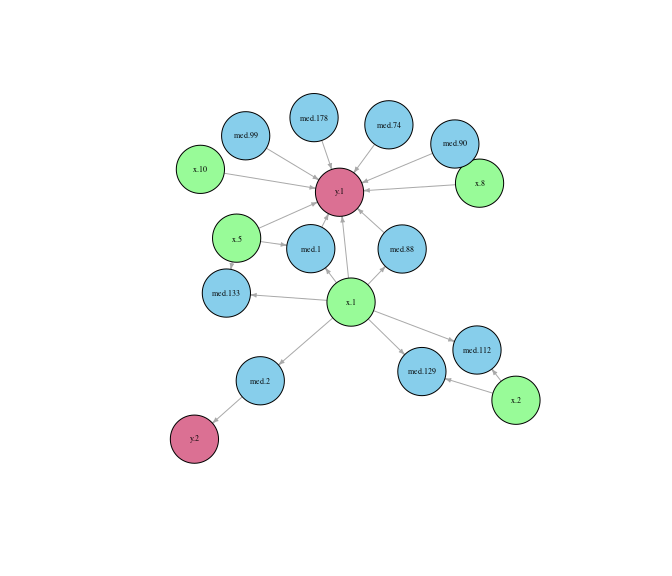## Lavaan sem: Estimate parameters of seleted model without imposing penalty

Like methods for regmed, we provide parallel methods for mvregmed that can be used to setup and fit models with lavaan sem. The 4 key steps are:

• determine edges with mvregmed.edges
• create the model with mvregmed.lavaan.model
• setup the data with mvregmed.lavaan.dat
• fit model with sem
mvmod <- mvregmed.lavaan.model(mvfit.edges, mvfit.best)
mvdat <- mvregmed.lavaan.dat(x, med, y)

mvfit.lavaan <- sem(model = mvmod, data = mvdat)

summary.lavaan(mvfit.lavaan)
       lhs op     rhs    est    se      z pvalue ci.lower ci.upper
1    med.1  ~     x.1  0.476 0.086  5.552  0.000    0.308    0.643
2    med.1  ~     x.5  0.174 0.085  2.041  0.041    0.007    0.341
3    med.2  ~     x.1  0.473 0.075  6.283  0.000    0.325    0.620
4   med.88  ~     x.1 -0.196 0.090 -2.172  0.030   -0.373   -0.019
5  med.112  ~     x.1 -0.164 0.106 -1.545  0.122   -0.373    0.044
6  med.112  ~     x.2 -0.141 0.105 -1.338  0.181   -0.348    0.066
7  med.129  ~     x.1  0.175 0.108  1.629  0.103   -0.036    0.386
8  med.129  ~     x.2  0.160 0.106  1.506  0.132   -0.048    0.368
9  med.133  ~     x.1  0.157 0.097  1.619  0.105   -0.033    0.346
10 med.133  ~     x.5  0.219 0.094  2.320  0.020    0.034    0.404
11     y.1  ~     x.1  0.280 0.102  2.745  0.006    0.080    0.480
12     y.1  ~     x.5  0.099 0.082  1.203  0.229   -0.062    0.261
13     y.1  ~     x.8  0.122 0.091  1.342  0.180   -0.056    0.299
14     y.1  ~    x.10 -0.248 0.079 -3.129  0.002   -0.403   -0.093
15     y.1  ~   med.1  0.317 0.089  3.557  0.000    0.143    0.492
16     y.1  ~  med.74 -0.140 0.076 -1.849  0.064   -0.288    0.008
17     y.1  ~  med.88 -0.114 0.075 -1.527  0.127   -0.261    0.032
18     y.1  ~  med.90  0.124 0.072  1.725  0.085   -0.017    0.265
19     y.1  ~  med.99 -0.158 0.072 -2.181  0.029   -0.299   -0.016
20     y.1  ~ med.178 -0.120 0.077 -1.569  0.117   -0.271    0.030
21     y.2  ~   med.2  0.399 0.093  4.267  0.000    0.216    0.582
22     y.1 ~~     y.1  0.468 0.066  7.071  0.000    0.338    0.598
23     y.2 ~~     y.2  0.820 0.116  7.071  0.000    0.593    1.047
24     y.1 ~~     y.2 -0.100 0.063 -1.594  0.111   -0.223    0.023

# Interpretation of lavaan output

The parameters alpha, beta, and delta are determined by regression models indicated by the tilde ($$\sim$$) operator, while variance and covariance parameters are indicated by the double tilder ($$\sim\sim$$) operator. Note that the covariance matrices for x and mediator are assumed fixed based on the values returned from regemd and mvregmed, so only variances and covariances for outcome y are provided. Also note that the z values and p-values from lavaan are marginal effects, not from a joint test of model parameters.